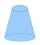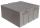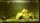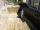Fractions + body volume - examples

1. Ratio of volumesIf the heights of two cylindrical drums are in the ratio 7:8 and their base radii are in the ratio 4:3. What is the ratio of their volumes?The radius of the sphere we reduce by 1/3 of the original radius. How much percent does the volume and surface of the sphere change?
3. Cube in a sphereThe cube is inscribed in a sphere with volume 6116 cm3. Determine the length of the edges of a cube.
4. Cone A2VSurface of cone in the plane is a circular arc with central angle of 126° and area 415 cm2. Calculate the volume of a cone.
5. ConeCircular cone of height 15 cm and volume 5699 cm3 is at one-third of the height (measured from the bottom) cut by a plane parallel to the base. Calculate the radius and circumference of the circular cut.
6. 2x coneCircular cone height 84 cm was cut plane parallel with base. Volume of these two small cones is the same. Calculate the height of the smaller cone.
7. Mystery of stereometrieTwo regular tetrahedrons have surfaces 88 cm2 and 198 cm2. In what ratio is their volumes? Write as a fraction and as a decimal rounded to 4 decimal places.
8. Stones in aquariumIn an aquarium with a length 2 m; width 1.5 m and a depth of 2.5 m is a water level up to three-quarters of the depth. Can we place stones with a volume of 2 m3 into the aquarium without water being poured out?
9. Concrete tankHow many m3 of concrete is in the tank shape of a cuboid with dimensions of 2000 cm and 5.6 meters and 70 dm, if the concrete takes up 3/7 of the tank?
10. TankThe tank bottom has dimensions of 1.5 m and 3 2/6 m. The tank is 459.1 hl of water. How high is the water surface?
11. ExcavationExcavation for the base of the cottage 4.5 m x 3.24 m x 60 cm. The excavated soil will increase its volume by one-quarter. Calculate the volume of excavated soil.
12. Stones in aquariumIn an aquarium with a length of 2 m, 1.5 m wide and 2.5 m deep, the water is up to three-quarters of the depth. Can we place 2m cubic meters of stones in the aquarium without spilling water? (0 = no, 1 = yes)
13. Cube, cuboid, and sphereVolumes of a cube and a cuboid are in ratio 3: 2. Volumes of sphere and cuboid are in ratio 1: 3. In what ratio are the volumes of cube, cuboid, and sphere?
14. The tankThe tank is full up to 4/5 of the total height and contains 240 hl of water. The area of the base is 6 square meters. What is the height of the tank?
15. RainfallA rectangular garden of 25m in length and width 20m in width fall 4mm of water. Express by a fraction in basic form what part of the 60-hectolitre tank we would fill with this water.
16. SeawaterSeawater has a density of 1025 kg/m3, ice 920 kg/m3. 8 liters of seawater froze and created a cube. Calculate the size of the cube edge.
17. Cube cutIn the ABCDA'B'C'D'cube, it is guided by the edge of the CC' a plane witch dividing the cube into two perpendicular four-sided and triangular prisms, whose volumes are 3:2. Determine in which ratio the edge AB is divided by this plane.
18. BucketThe bucket half filled with water weighs 5.55 kg, the full bucket weighs 9.85 kg. How much does the bucket weigh?
19. HectolitersHow deep is the pool if there are 2025 hectoliters of water and the bottom dimensions are a = 15 meters b = 7,5 meters and the water level is up to 9/10 (nine-tenths) of height.

Do you have an interesting mathematical example that you can't solve it? Enter it, and we can try to solve it.

To this e-mail address, we will reply solution; solved examples are also published here. Please enter e-mail correctly and check whether you don't have a full mailbox.

Need help calculate sum, simplify or multiply fractions? Try our fraction calculator.